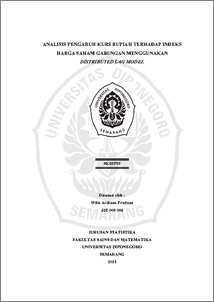# ANALISIS PENGARUH KURS RUPIAH TERHADAP INDEKS HARGA SAHAM GABUNGAN MENGGUNAKAN DISTRIBUTED LAG MODEL

Pradana, Wilis Ardiana (2016) ANALISIS PENGARUH KURS RUPIAH TERHADAP INDEKS HARGA SAHAM GABUNGAN MENGGUNAKAN DISTRIBUTED LAG MODEL. Undergraduate thesis, FSM Universitas Diponegoro.Preview
PDF
3438Kb

## Abstract

Analysis of distributed lag Statistics is a branch of science that discuss the case of time series data. The method can be used in a distributed lag analysis there are two Koyck Method and Almon Method. At Koyck Method of regression coefficients are assumed to have the same sign and decreases geometrically. In this method there is the dependent variable at a time ago as the independent variable so that the equation is autoregressive. By using this method to analyze the effect of the exchange rate against IHSG. Data used were 35 data. The results showed that the regression coefficient does not decrease geometrically, so that Koyck Method can not be used to resolve this case. To resolve this case, the used Almon Method. In the Almon Method assumed regression coefficient can be approximated by a polynomial has degree. Before applying this method to be specified maximum length of lag and the degree polynomial. To determine performed several experiments using lag length and degree polynomial different. Through these experiments the best results are obtained with a lag of three and a maximum length of second degree polynomials. The results indicate that the effect of the exchange rate against IHSG inversely. Keywords: Distributed Lag, Koyck Method, Almon Method, Autoregressive, Lag, Polynomial.

Item Type: Thesis (Undergraduate) H Social Sciences > HA Statistics Faculty of Science and Mathematics > Department of Statistics 49856 Mr Hasbi Yasin 24 Aug 2016 09:53 24 Aug 2016 09:53

Repository Staff Only: item control page